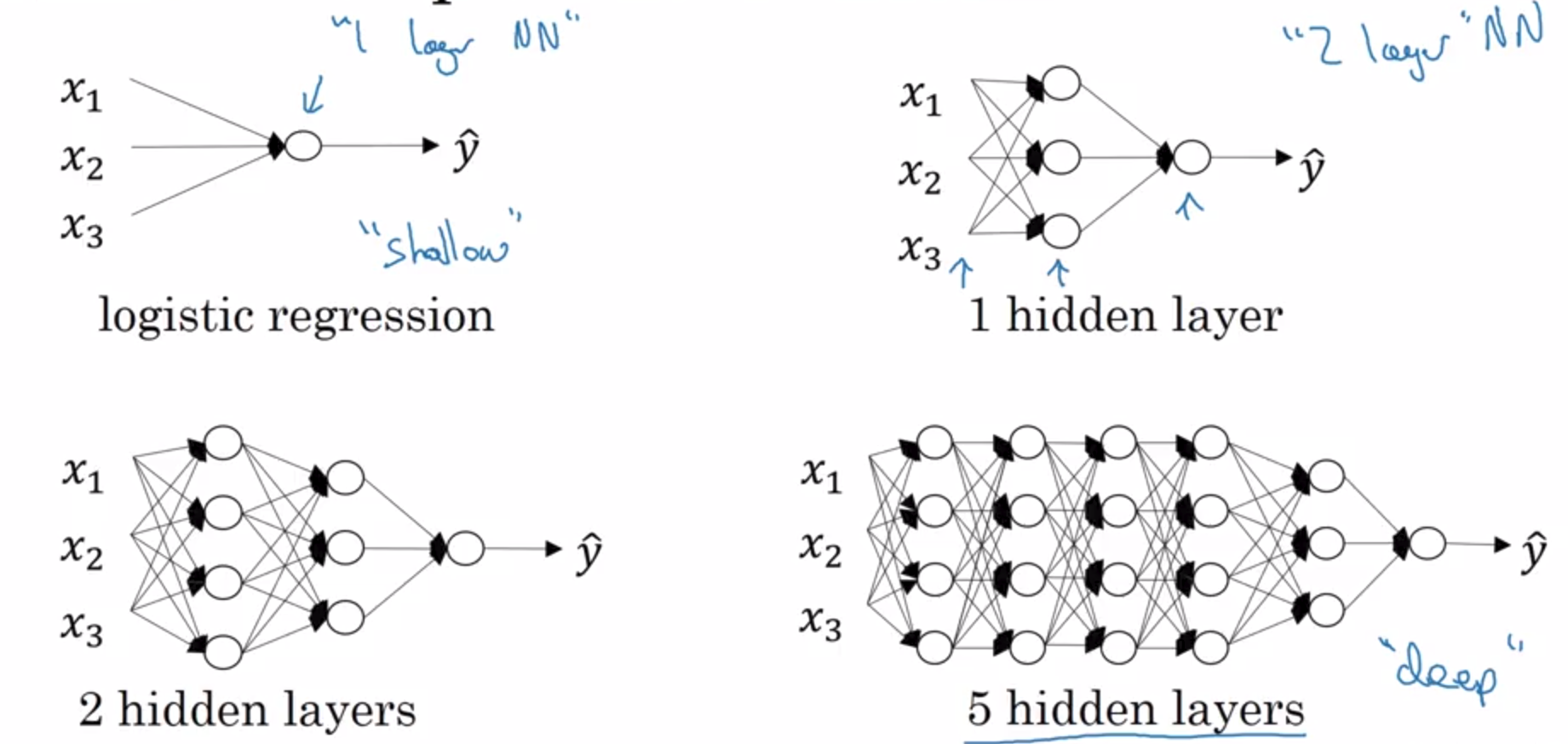# What is Deep Neural Network?as you can see, from the past 3 courses we have explored logistic regression as a shallow network.

## Notations

Notation Description
$l$ number of layers
$n$ number of hidden units
$n^{[l}$ number of hidden units at layer $l$
$a^{[l]}$ activations in layer $l$
$a^{[l]} = g^{[l]}(z^{[l]})$ activations in layer $l$
$W^{[l]}$ weights in layer $l$ for $z^{[l]}$

## Forward Propagation in Deep Network

basically same from before, lol# Getting your Matrix Dimension Right

to sum up:

Variable Shape / Dimension
$w^{[l]}$ $(n^{[l]}, n^{[l-1]})$
$b^{[l]}$ $(n^{[l]}, 1)$
$dw^{[l]}$ $(n^{[l]}, n^{[l-1]})$
$db^{[l]}$ $(n^{[l]}, 1)$

dimension of $z^{[l]}$ should be same as $a^{[l]}$

# Why Deep Neural Networks Works?

Circuit theory

There are functions you can compute with a “small” L-layer deep neural network that shallower networks require exponentially more hidden units to compute

means: if we try to compute a function $\hat y$ with depth $n$, and try to compute the same function with shallower network say with depth $n - x$, we might ended up need to add exponentially more hidden units (not layers), for example we might have to add $2^{x}$ more units to the current.

Deep learning is just branding!Start with logistic regression, then 1 hidden layer, then 2 hidden layer first!

Written on January 13, 2019